JMSLTM Numerical Library 7.2.0
com.imsl.stat

## Class InvCdf

• ### Method Summary

Methods
Modifier and Type Method and Description
`static double` ```beta(double p, double pin, double qin)```
Evaluates the inverse of the beta cumulative probability distribution function.
`static double` ```chi(double p, double df)```
Evaluates the inverse of the chi-squared cumulative probability distribution function.
`static int` ```discreteUniform(double p, int n)```
Returns the inverse of the discrete uniform cumulative probability distribution function.
`static double` ```exponential(double p, double scale)```
Evaluates the inverse of the exponential cumulative probability distribution function.
`static double` ```extremeValue(double p, double mu, double beta)```
Returns the inverse of the extreme value cumulative probability distribution function.
`static double` ```F(double p, double dfn, double dfd)```
Returns the inverse of the F cumulative probability distribution function.
`static double` ```gamma(double p, double a)```
Evaluates the inverse of the gamma cumulative probability distribution function.
`static double` ```geometric(double r, double pin)```
Returns the inverse of the discrete geometric cumulative probability distribution function.
`static double` ```logistic(double p, double mu, double s)```
Returns the inverse of the logistic cumulative probability distribution function.
`static double` ```logNormal(double p, double mu, double sigma)```
Returns the inverse of the standard lognormal cumulative probability distribution function.
`static double` ```noncentralBeta(double p, double shape1, double shape2, double lambda)```
Evaluates the inverse of the noncentral beta cumulative distribution function (CDF).
`static double` ```noncentralchi(double p, double df, double alam)```
Evaluates the inverse of the noncentral chi-squared cumulative probability distribution function.
`static double` ```noncentralF(double p, double dfn, double dfd, double lambda)```
Evaluates the inverse of the noncentral F cumulative distribution function (CDF).
`static double` ```noncentralstudentsT(double p, int idf, double delta)```
Evaluates the inverse of the noncentral Student's t cumulative probability distribution function.
`static double` `normal(double p)`
Evaluates the inverse of the normal (Gaussian) cumulative probability distribution function.
`static double` ```Pareto(double p, double xm, double k)```
Returns the inverse of the Pareto cumulative probability density function.
`static double` ```Rayleigh(double p, double alpha)```
Returns the inverse of the Rayleigh cumulative probability distribution function.
`static double` ```studentsT(double p, double df)```
Returns the inverse of the Student's t cumulative probability distribution function.
`static double` ```uniform(double p, double aa, double bb)```
Returns the inverse of the uniform cumulative probability distribution function.
`static double` ```Weibull(double p, double gamma, double alpha)```
Returns the inverse of the Weibull cumulative probability distribution function.
• ### Methods inherited from class java.lang.Object

`clone, equals, finalize, getClass, hashCode, notify, notifyAll, toString, wait, wait, wait`
• ### Method Detail

• #### beta

```public static double beta(double p,
double pin,
double qin)```
Evaluates the inverse of the beta cumulative probability distribution function.

Method `beta` evaluates the inverse distribution function of a beta random variable with parameters `pin` and `qin`, that is, with P = p, p = pin, and q = qin, it determines x (equal to `beta (p, pin, qin)`), such that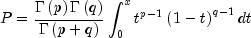where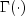is the gamma function. The probability that the random variable takes a value less than or equal to x is P.

Parameters:
`p` - a `double`, the probability for which the inverse of the beta CDF is to be evaluated.
`pin` - a `double`, the first beta distribution parameter.
`qin` - a `double`, the second beta distribution parameter.
Returns:
a `double`, the probability that a beta random variable takes a value less than or equal to this returned value is `p`.
Example
• #### chi

```public static double chi(double p,
double df)```
Evaluates the inverse of the chi-squared cumulative probability distribution function.

Method `chi` evaluates the inverse distribution function of a chi-squared random variable with `df` degrees of freedom, that is, with P = p and v = df, it determines x (equal to `chi(p, df)`), such that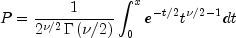whereis the gamma function. The probability that the random variable takes a value less than or equal to x is P.

For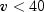, `chi` uses bisection, if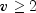or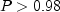, or regula falsi to find the point at which the chi-squared distribution function is equal to P. The distribution function is evaluated using `chi`.

For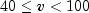, a modified Wilson-Hilferty approximation (Abramowitz and Stegun 1964, equation 26.4.18) to the normal distribution is used, and `normal` is used to evaluate the inverse of the normal distribution function. For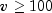, the ordinary Wilson-Hilferty approximation (Abramowitz and Stegun 1964, equation 26.4.17) is used.

Parameters:
`p` - a `double` scalar value representing the probability for which the inverse chi-squared function is to be evaluated.
`df` - a `double` scalar value representing the number of degrees of freedom. This must be at least 0.5.
Returns:
a `double` scalar value. The probability that a chi-squared random variable takes a value less than or equal to this returned value is `p`.
Example
• #### discreteUniform

```public static int discreteUniform(double p,
int n)```
Returns the inverse of the discrete uniform cumulative probability distribution function.
Parameters:
`p` - a `double` scalar value representing the probability for which the inverse discrete uniform function is to be evaluated
`n` - an `int` scalar value representing the upper limit of the discrete uniform distribution
Returns:
an `int` scalar value. The probability that a discrete uniform random variable takes a value less than or equal to this returned value is `p`.
• #### exponential

```public static double exponential(double p,
double scale)```
Evaluates the inverse of the exponential cumulative probability distribution function.

Method `exponential` evaluates the inverse distribution function of a gamma random variable with scale parameter =b and shape parameter a=1.0, that is, it determines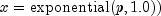, such that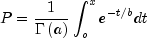whereis the gamma function. The probability that the random variable takes a value less than or equal to x is P. See the documentation for routine `gamma` for further discussion of the gamma distribution.

`exponential` uses bisection and modified regula falsi to invert the distribution function, which is evaluated using method `gamma`.

Parameters:
`p` - a `double` scalar value representing the probability at which the function is to be evaluated.
`scale` - a `double` scalar value representing the scale parameter.
Returns:
a `double` scalar value. The probability that an exponential random variable takes a value less than or equal to this returned value is `p`.
Example
• #### extremeValue

```public static double extremeValue(double p,
double mu,
double beta)```
Returns the inverse of the extreme value cumulative probability distribution function.
Parameters:
`p` - a `double` scalar value representing the probability for which the inverse extreme value function is to be evaluated.
`mu` - a `double` scalar value representing the location parameter.
`beta` - a `double` scalar value representing the scale parameter.
Returns:
a `double` scalar value. The probability that an extreme value random variable takes a value less than or equal to this returned value is `p`.
• #### F

```public static double F(double p,
double dfn,
double dfd)```
Returns the inverse of the F cumulative probability distribution function.

Method `F` evaluates the inverse distribution function of a Snedecor's F random variable with `dfn` numerator degrees of freedom and `dfd` denominator degrees of freedom. The function is evaluated by making a transformation to a beta random variable and then using `beta`. If X is an F variate with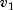and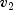degrees of freedom and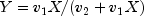, then Y is a beta variate with parameters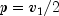and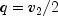. If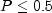, `F` uses this relationship directly, otherwise, it also uses a relationship between X random variables that can be expressed as follows, using `f`, which is the F cumulative distribution function: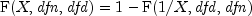Parameters:
`p` - a `double`, the probability for which the inverse of the F distribution function is to be evaluated. Argument p must be in the open interval (0.0, 1.0).
`dfn` - a `double`, the numerator degrees of freedom. It must be positive.
`dfd` - a `double`, the denominator degrees of freedom. It must be positive.
Returns:
a `double`, the probability that an F random variable takes a value less than or equal to this returned value is `p`.
Example
• #### gamma

```public static double gamma(double p,
double a)```
Evaluates the inverse of the gamma cumulative probability distribution function.

Method `gamma` evaluates the inverse distribution function of a gamma random variable with shape parameter a, that is, it determines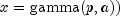, such that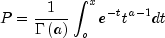whereis the gamma function. The probability that the random variable takes a value less than or equal to x is P. See the documentation for routine `gamma` for further discussion of the gamma distribution.

`gamma` uses bisection and modified regula falsi to invert the distribution function, which is evaluated using method `gamma`.

Parameters:
`p` - a `double` scalar value representing the probability at which the function is to be evaluated.
`a` - a `double` scalar value representing the shape parameter. This must be positive.
Returns:
a `double` scalar value. The probability that a gamma random variable takes a value less than or equal to this returned value is `p`.
Example
• #### geometric

```public static double geometric(double r,
double pin)```
Returns the inverse of the discrete geometric cumulative probability distribution function.
Parameters:
`r` - a `double` scalar value representing the probability for which the inverse geometric function is to be evaluated
`pin` - an `int` scalar value representing the probability parameter for each independent trial (the probability of success for each independent trial).
Returns:
a `double` scalar value. The probability that a geometric random variable takes a value less than or equal to this returned value is the input probability, `r`.
• #### logistic

```public static double logistic(double p,
double mu,
double s)```
Returns the inverse of the logistic cumulative probability distribution function.
Parameters:
`p` - a `double` scalar value representing the probability for which the inverse logistic function is to be evaluated.
`mu` - a `double` scalar value representing the location parameter,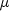.
`s` - a `double` scalar value representing the scale parameter.
Returns:
a `double` scalar value. The probability that a logistic random variable takes a value less than or equal to this returned value is `p`.
• #### logNormal

```public static double logNormal(double p,
double mu,
double sigma)```
Returns the inverse of the standard lognormal cumulative probability distribution function.
Parameters:
`p` - a `double` scalar value representing the probability for which the inverse lognormal function is to be evaluated.
`mu` - a `double` scalar value representing the location parameter.
`sigma` - a `double` scalar value representing the shape parameter. `sigma` must be a positive.
Returns:
a `double` scalar value. The probability that a standard lognormal random variable takes a value less than or equal to this returned value is `p`.
• #### noncentralBeta

```public static double noncentralBeta(double p,
double shape1,
double shape2,
double lambda)```
Evaluates the inverse of the noncentral beta cumulative distribution function (CDF).

If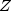is a noncentral chi-square random variable with noncentrality parameter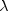and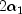degrees of freedom, and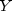is a chi-square random variable with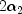degrees of freedom which is statistically independent of, then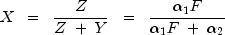is a noncentral beta-distributed random variable and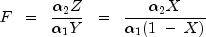is a noncentral F-distributed random variable. The CDF for noncentral beta variable X can thus be simply defined in terms of the noncentral F CDF: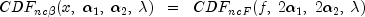where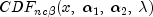is the noncentral beta CDF with= `x`,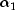= `shape1`,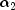= `shape2`, and noncentrality parameter= `lambda`;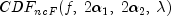is the noncentral F CDF with argument f, numerator and denominator degrees of freedomandrespectively, and noncentrality parameter; and: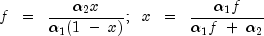(See documentation for class `Cdf` method `noncentralF` for a discussion of how the noncentral F CDF is defined and calculated.)

Method `noncentralBeta` evaluates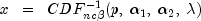by first evaluating: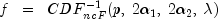and then solving for x using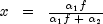. (See documentation for class `Cdf` method `noncentralF` for a discussion of how the inverse noncentral F CDF is calculated.)

Parameters:
`p` - a `double` scalar value representing the probability for which the inverse of the noncentral beta cumulative distribution function is to be evaluated. `p` must be non-negative and less than or equal to one.
`shape1` - a `double` scalar value representing the first shape parameter. `shape1` must be positive.
`shape2` - a `double` scalar value representing the second shape parameter. `shape2` must be positive.
`lambda` - a `double` scalar value representing the noncentrality parameter. `lambda` must be nonnegative.
Returns:
a `double` scalar value representing the inverse of the noncentral beta distribution function evaluated at `p`. The probability that a noncentral beta random variable takes a value less than or equal to `noncentralBeta` is `p`.
• #### noncentralchi

```public static double noncentralchi(double p,
double df,
double alam)```
Evaluates the inverse of the noncentral chi-squared cumulative probability distribution function.

Method `noncentralchi` evaluates the inverse distribution function of a noncentral chi-squared random variable with `df` degrees of freedom and noncentrality parameter `alam`, that is, with P = p,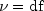, and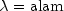, it determines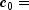`noncentralchi(p, df, alam)`), such that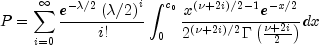whereis the gamma function. The probability that the random variable takes a value less than or equal to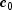is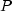.

Method `noncentralchi` uses bisection and modified regula falsi to invert the distribution function, which is evaluated using `noncentralchi`. See `noncentralchi` for an alternative definition of the noncentral chi-squared random variable in terms of normal random variables.

Parameters:
`p` - a `double` scalar value representing the probability for which the inverse noncentral chi-squared distribution function is to be evaluated. `p` must be in the open interval (0.0, 1.0).
`df` - a `double` scalar value representing the number of degrees of freedom. This must be at least 0.5. but less than or equal to 200,000.
`alam` - a `double` scalar value representing the noncentrality parameter. This must be nonnegative, and `alam + df ` must be less than or equal to 200,000.
Returns:
a `double` scalar value. The probability that a noncentral chi-squared random variable takes a value less than or equal to this returned value is `p`.
Example
• #### noncentralF

```public static double noncentralF(double p,
double dfn,
double dfd,
double lambda)```
Evaluates the inverse of the noncentral F cumulative distribution function (CDF).

If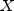is a noncentral chi-square random variable with noncentrality parameterand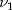degrees of freedom, andis a chi-square random variable with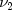degrees of freedom which is statistically independent of, then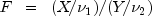is a noncentral F-distributed random variable whose CDF is given by: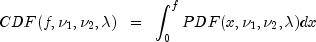where the probability density function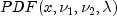is given by: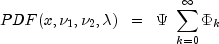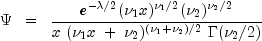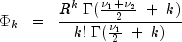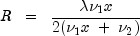whereis the gamma function,= `dfn`,= `dfd`,= `lambda`, and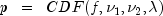is the probability that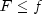.

Method `noncentralF` evaluates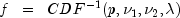Method `noncentralF` uses bisection and modified regula falsi search algorithms to invert the distribution function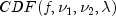, which is evaluated using method `noncentralF`. For sufficiently small p, an accurate approximation of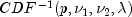can be used which requires no such inverse search algorithms.

Parameters:
`p` - a `double` scalar value representing the probability for which the inverse of the noncentral F cumulative distribution function is to be evaluated. `p` must be non-negative and less than one.
`dfn` - a `double` scalar value representing the number of numerator degrees of freedom. `dfn` must be positive.
`dfd` - a `double` scalar value representing the number of denominator degrees of freedom. `dfd` must be positive.
`lambda` - a `double` scalar value representing the noncentrality parameter. `lambda` must nonnegative.
Returns:
a `double` scalar value representing the inverse of the noncentral F distribution function evaluated at p. The probability that a noncentral F random variable takes a value less than or equal to `noncentralF(p, dfn, dfd, lambda)` is `p`.
• #### noncentralstudentsT

```public static double noncentralstudentsT(double p,
int idf,
double delta)```
Evaluates the inverse of the noncentral Student's t cumulative probability distribution function.

Method `noncentralstudentsT` evaluates the inverse distribution function of a noncentral t random variable with `idf` degrees of freedom and noncentrality parameter `delta`; that is, with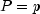,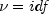,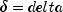, it determines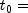`noncentralstudentsT(p, idf, delta)`), such that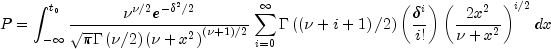whereis the gamma function. The probability that the random variable takes a value less than or equal to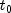is `P`. See `noncentralstudentsT` for an alternative definition in terms of normal and chi-squared random variables. The method `noncentralstudentsT` uses bisection and modified regula falsi to invert the distribution function, which is evaluated using `noncentralstudentsT`.

Parameters:
`p` - a `double` scalar value representing the probability for which the function is to be evaluated.
`idf` - an `int` scalar value representing the number of degrees of freedom. This must be positive.
`delta` - a `double` scalar value representing the noncentrality parameter.
Returns:
a `double` scalar value. The probability that a noncentral Student's t random variable takes a value less than or equal to this returned value is `p`.
Example
• #### normal

`public static double normal(double p)`
Evaluates the inverse of the normal (Gaussian) cumulative probability distribution function.

Method `normal` evaluates the inverse of the distribution function,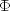, of a standard normal (Gaussian) random variable, that is, `normal`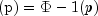, where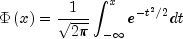The value of the distribution function at the point x is the probability that the random variable takes a value less than or equal to x. The standard normal distribution has a mean of 0 and a variance of 1.

Parameters:
`p` - a `double` scalar value representing the probability at which the function is to be evaluated.
Returns:
a `double` scalar value. The probability that a standard normal random variable takes a value less than or equal to this returned value is `p`.
Example
• #### Pareto

```public static double Pareto(double p,
double xm,
double k)```
Returns the inverse of the Pareto cumulative probability density function.
Parameters:
`p` - a `double` scalar value representing the probability for which the inverse Pareto function is to be evaluated.
`xm` - a `double` scalar value representing the scale parameter,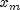.
`k` - a `double` scalar value representing the shape parameter.
Returns:
a `double` scalar value. The probability that a Pareto random variable takes on a value less than or equal to this returned value is `p`.
• #### Rayleigh

```public static double Rayleigh(double p,
double alpha)```
Returns the inverse of the Rayleigh cumulative probability distribution function.
Parameters:
`p` - a `double` scalar value representing the probability for which the inverse Rayleigh function is to be evaluated.
`alpha` - a `double` scalar value representing the scale parameter.
Returns:
a `double` scalar value. The probability that a Rayleigh random variable takes a value less than or equal to this returned value is `p`.
• #### studentsT

```public static double studentsT(double p,
double df)```
Returns the inverse of the Student's t cumulative probability distribution function.

`studentsT` evaluates the inverse distribution function of a Student's t random variable with `df` degrees of freedom. Let v = df. If v equals 1 or 2, the inverse can be obtained in closed form, if v is between 1 and 2, the relationship of a t to a beta random variable is exploited and `beta` is used to evaluate the inverse; otherwise the algorithm of Hill (1970) is used. For small values of v greater than 2, Hill's algorithm inverts an integrated expansion in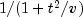of the t density. For larger values, an asymptotic inverse Cornish-Fisher type expansion about normal deviates is used.

Parameters:
`p` - a `double` scalar value representing the probability for which the inverse Student's t function is to be evaluated.
`df` - a `double` scalar value representing the number of degrees of freedom. This must be at least one.
Returns:
a `double` scalar value. The probability that a Student's t random variable takes a value less than or equal to this returned value is `p`.
Example
• #### uniform

```public static double uniform(double p,
double aa,
double bb)```
Returns the inverse of the uniform cumulative probability distribution function.
Parameters:
`p` - a `double` scalar value representing the probability for which the inverse uniform function is to be evaluated.
`aa` - a `double` scalar value representing the minimum value.
`bb` - a `double` scalar value representing the maximum value.
Returns:
a `double` scalar value. The probability that a uniform random variable takes a value less than or equal to this returned value is `p`.
• #### Weibull

```public static double Weibull(double p,
double gamma,
double alpha)```
Returns the inverse of the Weibull cumulative probability distribution function.
Parameters:
`p` - a `double` scalar value representing the probability for which the inverse Weibull function is to be evaluated.
`gamma` - a `double` scalar value representing the shape parameter.
`alpha` - a `double` scalar value representing the scale parameter.
Returns:
a `double` scalar value. The probability that a Weibull random variable takes a value less than or equal to this returned value is `p`.
JMSLTM Numerical Library 7.2.0